# State Space Analysis – 1 – 3

11. The eigenvalues of the state model are the same as the :

(a) open-loop poles
(b) closed-loop poles
(c) both the open-loop and closed-loop poles
(d) none of these

Explanation
No answer description available for this question. Let us discuss.

12. The state variable approach is applicable to :

(a) only linear time-invariant systems
(b) linear time-invariant as well as time-varying systems
(c) linear as well as nonlinear systems
(d) all systems

Explanation
No answer description available for this question. Let us discuss.

13.  Φ (s) is called the :

(a) state transition matrix
(b) resolution matrix
(c) resolvent matrix
(d) transfer matrix

Explanation
No answer description available for this question. Let us discuss.

14. Using state variables, an nth – order differential equation can be decomposed into :

(a) n number of first – order differential equations
(b) n/2 number of first – order diffferential equations
(c) unlimited number of first – order differential equations
(d) none of these

Explanation
No answer description available for this question. Let us discuss.

15. D is called the :

(a) system matrix
(b) transmission matrix
(c) transfer matrix
(d) output matrix

Explanation
No answer description available for this question. Let us discuss.

General Knowledge Books##-17% General Knowledge 2019 Price: INR 25.00 Was: INR 30.00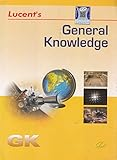-17% General Knowledge Price: INR 179.00 Was: INR 216.00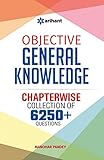-43% Objective General Knowledge Chapterwise Collection of 6250+ Questions Price: INR 169.00 Was: INR 295.00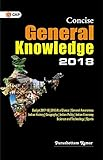Concise General Knowledge 2018 Price: INR 30.00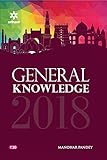General Knowledge 2018 (Old Edition) Price: Check on Amazon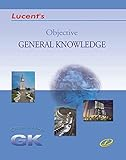-23% Objective General Knowledge Price: INR 302.00 Was: INR 390.00 ‹›

Be a Part of the New & Next

Share the Knowledge

Copy Protected by Chetan's WP-Copyprotect.